# GED Practice: Converting Units of Measurement

Today’s topic for GED practice: converting units of measurement. Measurement is an important skill on the GED exam. Both the Mathematical Reasoning and the Science subject tests may ask you to work with various units of measurement. You’ll need to understand how units of measure work and how to convert between them.

There are two common systems of measurement. The U.S. Standard System of Measurement includes units such as inches, feet, and miles. Elsewhere across the globe, the metric system is used. The metric system includes units such as centimeters, meters, and kilometers.

## Why Do We Need to Convert Units of Measurement?

Standardized units allow us to measure the physical world, including dimensions such as length, mass, and volume. For smaller objects we need smaller units and vice versa. You wouldn’t, after all, want to measure the distance to the moon in centimeters. Conversely, it doesn’t make sense to measure the length of a room in kilometers.

As the frame of reference changes, it’s common to need to convert units. For example, one student might drink a cup of milk during school lunch, but an entire school might drink 35 gallons of milk a day. The basic concepts behind converting are the same whether you are using the U.S. or the metric system.

## The U.S. Standard System

### Volume

Volume, or capacity, is a measure of how much space something takes up (often a liquid). There are five basic units for measuring capacity in the U.S. Standard System:

Unit of Measurement Conversions
fluid ounces (fl. oz.)
cup 8 fl. oz. = 1 cup
pint 16 fl. oz. = 2 cups = 1 pint
quart 32 fl. oz. = 4 cups = 2 pints = 1 quart
gallon 128 fl. oz. = 16 cups = 8 pints = 4 quarts = 1 gallon

### Mass

Mass is a measure of how big something is. The U.S. system has three basic units for measuring weight:

Unit of Measurement Conversions
ounce (oz.)
pound (lb.) 16 oz. = 1 lb.
ton (T) 32,000 oz. = 2,000 lbs. = 1 T

While technically mass is a measure of how much matter something has, usually the terms mass and weight are used interchangeably. Weight is the measure of how much force gravity has upon an object (in other words, how heavy it is). This is the measurement commonly thought of when using ounces, pounds, and tons.

Also, don’t confuse fluid ounces and ounces. Fluid ounces measure volume; ounces measure mass or weight. These two units are not interchangeable.

### Length

Length measures distance. The U.S. system has four basic units for measuring length:

Unit of Measurement Conversions
inch
feet12 inches = 1 foot
yard 36 inches = 3 feet = 1 yard
mile 5,280 feet = 1,760 yards = 1 mile

### Converting Among U.S. Units

To convert among units in the U.S. system, the first thing you need to know is the relationship between units. It is helpful to memorize the charts above for this purpose.

To convert between units, multiply by a unit rate of conversion. The unit rate tells you how many smaller units equal 1 larger unit. Usually you want to set up the unit rate as a fraction. For example:Now that you know the unit rate, multiply it by the number of units you have. For example, if you are converting 5 yards to inches, you would calculate:Make sure you are cancelling units correctly when you convert. Remember that if you have a unit on the top and bottom of a fraction, those units cancel out. If you cannot cancel units, you have not set up your ratio correctly. To fix it, simply swap the numerator and denominator, and try multiplying again.

The example above shows you how to convert from a larger unit to a smaller unit. Converting from a smaller unit to a larger unit involves the same process, except that you will set up your unit rate with larger unit on top instead of on the bottom. For example:To convert, just multiply the number of units you are converting by the unit rate. To simplify, you will need to divide. For example, if you are converting 84 inches to feet, you would calculate:To check if you set up your calculation correctly, look at whether the number of units increased or decreased. When you convert to a smaller unit, you will end up with a higher number. Think about it: you need more of a smaller unit to fill up the same amount of space as a larger unit. So, if you are going from a larger unit to a smaller unit, your amount of units will go up. Likewise, if you are converting from a smaller unit to a larger unit, the number of units will go down.

## The Metric System

The metric system measures the same dimensions that the U.S. system does, except it has different units. In fact, each type of measurement (volume, mass/weight, and length) has only 1 basic unit:

Measurement Unit
volume liters
mass grams
length meter

### Converting Among Metric Units

The metric system is based on units of 10, which makes converting much easier than converting among U.S. units, as long as you have your prefixes memorized.

Prefix Number of Basic Units
giga- 1,000,000,000
mega- 1,000,000
kilo-1,000
hecto-100
deka-10
BASIC UNIT
deci-110
centi-1100
milli- 11,000
micro-11,000,000
nano-11,000,000,000

To use a common example, 1 centimeter is one hundredth of a meter. That means 100 centimeters = 1 meter. Using this logic, you can deduce that 1 micrometer is 1 millionth of meter; thus, 1 million micrometers = 1 meter.

Converting from the basic unit (that is, gram, meter, or liter) to any other unit is fairly easy—that is why when converting from any unit in the metric system to another, it is best to use a two-step process in which you first convert the units you start with to the basic unit, and then convert the basic unit to the desired unit.

#### Step One: Converting to the Basic Unit

Say you need to convert 48.5 kilograms to milligrams. It might be difficult to conceptualize this conversion, because you begin with a unit that is larger than grams, and you end up with a unit that is smaller than grams.

So first, to simplify things, convert 48.5 kilograms to grams. Thinking about the prefixes above, you should know that 1,000 grams = 1 kilogram. Use this as your unit rate and multiply as you did with U.S. Standard units.#### Step Two: Converting to the Desired Unit

Now that you have converted to the basic unit, set up another ratio showing the unit rate for the desired unit. In this case, you are converting the number of grams to milligrams. Remember, there are 1,000 milligrams in 1 gram. Since you are converting again to a smaller unit, multiply the number of grams you are converting by the unit rate: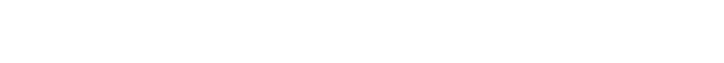So, 48.5 grams equals 48,500,000 milligrams.

## Sample Problems

Try solving these problems for extra practice.

1. What is 3 tons converted to pounds?
a) 3,000 pounds
b) two-thirds pound
c) 6,000 pounds
d) one-third pound

2. What is 54 inches converted to yards?
a) 1.5 yards
b) 1,944 yards
c) .66 yards
d) 18 yards

3. What is 6,500 centimeters converted to kilometers?
a) .065 kilometers
b) .65 kilometers
c) 6.5 kilometers
d) 65 kilometers

4. What is 8.9 dekaliters converted to milliliters?
a) 89 milliliters
b) 890 milliliters
c) 8,900 milliliters
d) 89,000 milliliters

1. c) 6,000 pounds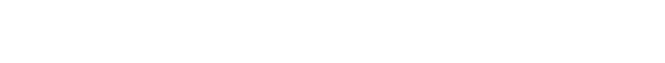2. a) 1.5 yards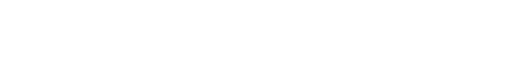3. a) .065 kilometers
First, convert 6,500 centimeters to meters: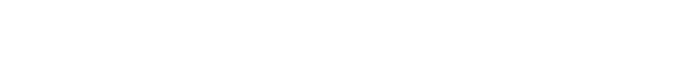Next, convert 65 meters to kilometers: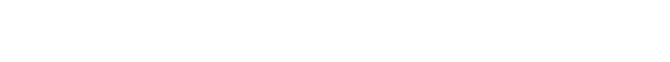4. d) 89,000 milliliters
First, convert 8.9 dekaliters to liters: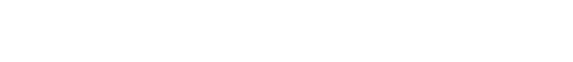Next, convert 89 liters to milliliters:## Next Steps

Feeling confident? Put your newly-honed skills to the test by trying out a practice test.

Want to know more about unit conversion? Khan Academy has some great resources, including lessons, videos, and practice questions.

For more GED prep, you should also check out our 5 Ways to Study for the GED Online.